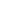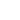AI

CIFAR-10之蛙探索不同的生成式架构

CPPN的架构限制了网络可能绘制的图像种类。不同的架构对应不同种类的生成图像，尽管这些架构都是在相似的数据集上训练的。``````H = tf.nn.tanh(U)

for i in range(1, self.net_depth_g):
H = tf.nn.tanh(fully_connected(H, n_network, self.model_name+'_g_tanh_'+str(i)))

output = tf.nn.sigmoid(fully_connected(H, self.c_dim, self.model_name+'_g_'+str(self.net_depth_g)))
````````````H = tf.nn.relu(U)

for i in range(1, self.net_depth_g):
H = tf.nn.tanh(fully_connected(H, n_network, self.model_name+'_g_tanh_'+str(i)))

output = tf.nn.sigmoid(fully_connected(H, self.c_dim, self.model_name+'_g_'+str(self.net_depth_g)))
````````````H = tf.nn.relu(U)

for i in range(1, self.net_depth_g):
H = tf.nn.tanh(fully_connected(H, n_network, self.model_name+'_g_tanh_'+str(i)))
H = tf.nn.relu(fully_connected(H, n_network, self.model_name+'_g_relu_'+str(i)))

output = tf.nn.sigmoid(fully_connected(H, self.c_dim, self.model_name+'_g_'+str(self.net_depth_g)))
``````

CIFAR-10 青蛙CIFAR-10中的图像尺寸为32x32像素。我们略加裁切，得到30x30像素的训练图像。此外，我们在训练中随机水平翻转图像，这样可以增加每个分类中数量较少的数据的有用程度（训练集与测试集中共有6000张青蛙图片）。

CIFAR-10的青蛙分类中的一些样本：CIFAR-10 卡车CIFAR-10 卡车30x30和1080x1080分辨率下的CPPN CIFAR卡车样本结论

• 将VAE编码器网络从一个简单的全连接双层softplus网络修改为一个能够以空间不变的方式更好地识别图像特征的更深的卷积神经网络。

• 在图像的离散余弦变换版本上训练网络，让网络生成新图像的离散余弦变换表示，然后再把表示转换成空间上的表示。

• 修改生成网络，以利用残差/随机层进行训练。让10层以上的网络学习已被证明非常具有挑战性。任何dropout处的尝试都会使网络生成非常模糊的图像。

• 将生成网络中的全连接层替换为稀疏连接层，或卷积神经网络类的连接。尝试使用DCGAN类的架构，不过生成网络起类似CPPN的作用。

评论游客2017-07-22

2018-01-19

2017-12-13

2017-12-08

不放过任何细节 “火眼金睛”Tiny Faces算法简介发布需求联系客服